# Translations of Encyclopedia about Mathematics

## Vector Calculus

Around 1884, German mathematician Hermann G. Grassmann (1809-1877) and Irish mathematician William R. Hamilton (1805-1865) founded vector mathematics independently of one another. Hamilton founded the concept of vectors, which have become essential tools of geometry and physical mathematics. Austrian mathematician Wilhelm J. E. Blaschke (1885-1962) introduced it into geometry and British physicist James Clerk Maxwell (1831-1879) into physics.

Scalars are quantities which are only general indications of numerical quantities, such as those describing temperature, mass or wave lengths and which can also indicate dimension. Contrary to this, vectors are quantities which, besides their numerical aspect, be it their dimensional or size aspect, also indicate direction, which is very important when describing for example speed or rotational moment. An abscissa which indicates direction and which can be shifted in parallel within a space or plain is considered a vector. Vectors lying in some plain have only two coordinates, while those lying in a three-dimensional space require three coordinates. In technical literature, coordinates can also be referred to as scalar components of vectors.

A vector is described as an arrow above two points, such as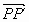, which describes the position of an abscissa between the points of P a P´. We refer to P as the point of origin and point P´ as the target point. The length and arrows are called the vector’s weight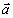, which we write as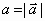. If the statement appears as: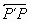, we are referring to inverse vectors. If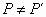, it is different from. If we have an entire class of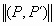, where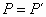, P = 0 and P` = 0, where the start and end point of a vector overlap, we are referring to a null vector. If for each vectorthere exists a substitute whose beginning point (P) lies at the origin of a coordinate system and whose arrow points to point P´, we are referring to a local vector (fixed vector) of point P´.

We refer to two vectors (P,P´) and (Q,Q´) as parallel if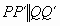,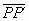=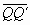with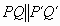. Therefore, two vectors are parallel if their dimensions and directions are the same. A class of parallel arrows is referred to as a vector,=. A unit vector is a vector whose absolute value equals 1, which we write as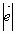=1. Free vectors are vectors which have a free or non-fixed starting point. The length of a vector is calculated from the coordinate:

P(x1,y1,z1); P´(x2,y2,z2) from which it follows that:

P=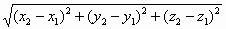The following basic operations can be performed with free vectors:

Addition:

If P(ax,ay,az) and P´(bx,by,bz) are two vectors, then a vector with coordinate (ax+bx,ay+by,az+bz) will be their combined sum and the vector a+b will result from the addition of vectors a and b. The addition of vectors is commutative and associative.

Subtraction:

This is the difference of a-b between vectors a and b if we add the vector (-b) to the vector a.

Multiplying by real numbers:

The vector P = (bx-ax, by-ay, bz-az) can be multiplied by any real number c by multiplying its length by the value c. If the value c is positive, the original direction remains intact; if negative, the direction reverses.

If: c>0 the direction will be same as the vector

c<0 the direction will be an opposite product to the direction of the vector

c=0 the product ca=0

ac = ca and if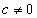, then=a

from which it follows that a and c are parallel vectors

if c=0 or a=0, then also ca=0
if a=b then also ca
if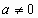and ca=da, then also c=d
if a and b are parallel to one another and neither of them are null-vectors.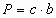; b=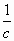a

If two vectors a and b are parallel, one of them may be considered a scalar multiplication of the other, and vice versa;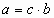when c =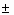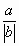. Positive signs apply if a and b are parallel to one another and negative if oriented in the opposite direction.

Angles Between Vectors

The product of the scalars of vectors a and b equals the product of their sizes using the cosine of the angle contained between them. The result is not a vector but rather a real number.

A unit vector e is assigned using e = b0 =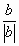, in which case: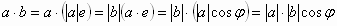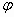is the angle of incline of both vectors 0°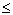180°

- ab=ba

- ab=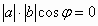if a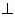b

- ab>0 if 0°90° when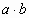<0 if 90° <180°

- a2=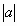2

- the scalar product of unit vectors e and e* : ee*=cosUsing this, it is possible to determine the angle between two abscissae.
Using coordinates of vectors, it is possible to convert operations with vectors back to operations with numbers.

Translating Dutch Hungarian Translations Hungarian Dutch Translating Swedish Czech Translations Czech Swedish Translating RussianEnter your search terms Submit search form

Copyright © KENAX, by Karel Kosman - All Rights Reserved Worldwide.